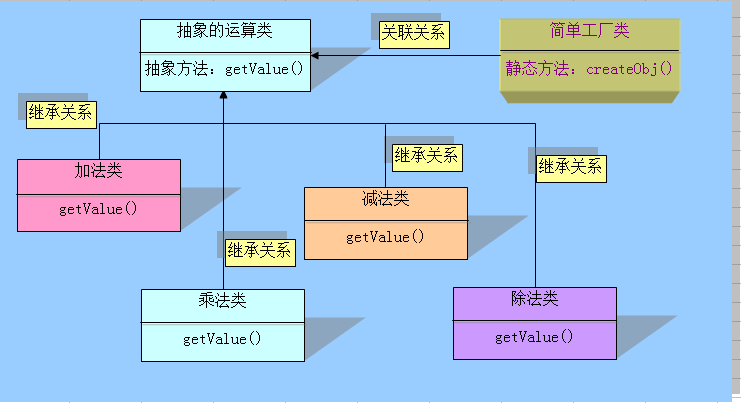# PHP 常用设计模式 (转载)

1.单例模式

php的应用主要在于数据库应用, 一个应用中会存在大量的数据库操作, 在使用面向对象的方式开发时, 如果使用单例模式, 则可以避免大量的new 操作消耗的资源,还可以减少数据库连接这样就不容易出现 too many connections情况。

``````/**
* 设计模式之单例模式
* \$_instance必须声明为静态的私有变量
* 构造函数必须声明为私有,防止外部程序new类从而失去单例模式的意义
* getInstance()方法必须设置为公有的,必须调用此方法以返回实例的一个引用
* ::操作符只能访问静态变量和静态函数
* new对象都会消耗内存
* 使用场景:最常用的地方是数据库连接。
* 使用单例模式生成一个对象后，该对象可以被其它众多对象所使用。
*/
class man
{
//保存例实例在此属性中
private static \$_instance;
//构造函数声明为private,防止直接创建对象
private function __construct()
{
echo '我被实例化了！';
}
//单例方法
public static function get_instance()
{
var_dump(isset(self::\$_instance));
if(!isset(self::\$_instance))
{
self::\$_instance=new self();
}
return self::\$_instance;
}
//阻止用户复制对象实例
private function __clone()
{
trigger_error('Clone is not allow',E_USER_ERROR);
}
function test()
{
echo("test");
}
}
// 这个写法会出错，因为构造方法被声明为private
//\$test = new man;
// 下面将得到Example类的单例对象
\$test = man::get_instance();
\$test = man::get_instance();
\$test->test();
// 复制对象将导致一个E_USER_ERROR.
//\$test_clone = clone \$test;``````

2.简单工厂模式

①抽象基类：类中定义抽象一些方法，用以在子类中实现

②继承自抽象基类的子类：实现基类中的抽象方法

③工厂类：用以实例化所有相对应的子类`````` /**
*
* 定义个抽象的类，让子类去继承实现它
*
*/
abstract class Operation{
//抽象方法不能包含函数体
abstract public function getValue(\$num1,\$num2);//强烈要求子类必须实现该功能函数
}
/**
* 加法类
*/
public function getValue(\$num1,\$num2){
return \$num1+\$num2;
}
}
/**
* 减法类
*/
class OperationSub extends Operation {
public function getValue(\$num1,\$num2){
return \$num1-\$num2;
}
}
/**
* 乘法类
*/
class OperationMul extends Operation {
public function getValue(\$num1,\$num2){
return \$num1*\$num2;
}
}
/**
* 除法类
*/
class OperationDiv extends Operation {
public function getValue(\$num1,\$num2){
try {
if (\$num2==0){
throw new Exception("除数不能为0");
}else {
return \$num1/\$num2;
}
}catch (Exception \$e){
echo "错误信息：".\$e->getMessage();
}
}
}``````

``````/**
* 求余类（remainder）
*
*/
class OperationRem extends Operation {
public function getValue(\$num1,\$num2){
return \$num1%\$num12;
}
}``````

`````` /**
* 工程类，主要用来创建对象
* 功能：根据输入的运算符号，工厂就能实例化出合适的对象
*
*/
class Factory{
public static function createObj(\$operate){
switch (\$operate){
case '+':
break;
case '-':
return new OperationSub();
break;
case '*':
return new OperationSub();
break;
case '/':
return new OperationDiv();
break;
}
}
}
\$test=Factory::createObj('/');
\$result=\$test->getValue(23,0);
echo \$result;``````

3.观察者模式

``````class MyObserver1 implements SplObserver {
public function update(SplSubject \$subject) {
echo __CLASS__ . ' - ' . \$subject->getName();
}
}
class MyObserver2 implements SplObserver {
public function update(SplSubject \$subject) {
echo __CLASS__ . ' - ' . \$subject->getName();
}
}
class MySubject implements SplSubject {
private \$_observers;
private \$_name;
public function __construct(\$name) {
\$this->_observers = new SplObjectStorage();
\$this->_name = \$name;
}
public function attach(SplObserver \$observer) {
\$this->_observers->attach(\$observer);
}
public function detach(SplObserver \$observer) {
\$this->_observers->detach(\$observer);
}
public function notify() {
foreach (\$this->_observers as \$observer) {
\$observer->update(\$this);
}
}
public function getName() {
return \$this->_name;
}
}
\$observer1 = new MyObserver1();
\$observer2 = new MyObserver2();
\$subject = new MySubject("test");
\$subject->attach(\$observer1);
\$subject->attach(\$observer2);``````

4.策略模式

``````interface IStrategy {
function filter(\$record);
}``````

``````class FindAfterStrategy implements IStrategy {
private \$_name;
public function __construct(\$name) {
\$this->_name = \$name;
}
public function filter(\$record) {
return strcmp ( \$this->_name, \$record ) = 0.5;
}
}``````

//主类

``````class UserList {
private \$_list = array ();
public function __construct(\$names) {
if (\$names != null) {
foreach ( \$names as \$name ) {
\$this->_list [] = \$name;
}
}
}
\$this->_list [] = \$name;
}
public function find(\$filter) {
\$recs = array ();
foreach ( \$this->_list as \$user ) {
if (\$filter->filter ( \$user ))
\$recs [] = \$user;
}
return \$recs;
}
}
\$ul = new UserList ( array (
"Andy",
"Jack",
"Lori",
"Megan"
) );
\$f1 = \$ul->find ( new FindAfterStrategy ( "J" ) );
print_r ( \$f1 );
\$f2 = \$ul->find ( new RandomStrategy () );
print_r ( \$f2 );  ``````